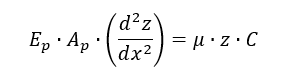# Settlement analysis under axial load for single piles

1.0 Introduction

Settlement prediction of single piles under axial loading is as important as the estimation of ultimate axial capacity which is the sum of ultimate total shaft resistance and end bearing resistance. Settlement control usually receives the most intention for geotechnical designers who usually have the difficulties in complying with the settlement requirements as specified in many design specifications or standards if there is no thorough understanding of the mechanism involved in the settlement analysis of single piles under axial loading.

It would be very useful to develop a powerful but simple tool that could predict the pile load settlement curve in addition to estimating axial pile capacity.

2.0 Background

The widely-used methods for predicting settlement under axial load for single pile can be categorized into three different types: (1) load transfer method; (2) elastic theory based method and (3) finite element or finite difference method.

The load transfer method was developed by Coyle and Reese (1966). In this method, the pile is divided into a number of segments. The movement of the individual pile segment at mid-height is estimated from load transfer versus pile movement curves (empirical t-z curves for shaft resistance and q-w curves for end bearing resistance) and the elastic axial deformation in an iterative manner for an assumed tip movement. The calculation proceeds up the length of the pile to obtain the load and displacement at the pile head. The pile load settlement curve can thus be constructed through a series of such calculations with different assumed tip movement values.

Elastic theory based method is adopted by Butterfield and Banerjee (1971), Randolph and Wroth (1978) and Poulos and Davis (1980). In this method, the pile is divided into a number of uniformly loaded elements and the solution is obtained by imposing the compatibility constraints between the displacement of the soil and the pile. The displacements of the pile are obtained by considering the compressibility of the pile under axial loading and the soil displacements in most cases are obtained by using Mindlin’s equations for the displacement of a soil mass caused by a loading within the mass. The limitation of the elasticity method lies in the basic assumptions that must be made – semi-infinite, elastic and isotropic solids. The actual ground conditions rarely satisfy the basic assumption of uniform and isotropic materials. In spite of the highly nonlinear stress-strain characteristics of soils, the only soil properties considered in the elasticity method are Young’s modulus E and the Poisson’s ratio, mu. The use of only two constants, E and mu, to represent soil characteristics is an oversimplification to allow the elasticity-based methods to work in conditions involving stratified soils with varying strengths and stiffness.

Finite element or finite difference method is considered as one of the most powerful approaches for the analysis of single piles and pile groups under axial loading. However, it is not commonly used in practice due to its high computational requirements.

3.0 Numerical Simulation of Load Transfer

Numerical simulation of the load transfer curves such as t-z curves for shaft resistance and q-w curves for end bearing resistance is a practical approach for the conditions where layered soil profiles and/or many potential load cases and trial designs need to be undertaken.A free body diagram of a single pile under axial loading is shown in the figure on the right. The pile is evenly divided into a number of pile segments.

The basic form of the differential equation is presented as below for calculating the settlement of single piles under axial loading. The differential equation is usually solved using the difference-equation technique.Where EpAp is axial stiffness of the pile, z is relative movement of the pile with reference to the soil at point z, C is circumference of the pile, u is the modulus from the load transfer curves for t-z and q-w relationships.

PileAXL program uses the load transfer method to estimate the settlement under axial load for single pile due to its simplicity and practicality. It can deal with any complex composition of soil layers with any nonlinear relationship of displacement versus shaft resistance or end bearing resistance. A recursive solution is adopted to solve the equation.

4.0 T-z curves for shaft resistance mobilization

A typical nonlinear T-Z load transfer curve used to simulate the mobilization of shaft resistance with displacement is shown in the figure below.The following table tabulates all the nonlinear T-Z load transfer curves implemented in PileAXL program for pile settlement calculation. Those t-z load transfer curves are widely used to simulate the mobilization of shaft resistance with vertical displacement.5.0 Q-w curves for end bearing resistance mobilization

Similar to t-z load transfer curves for modelling the mobilization of shaft resistance along the pile, q-w curves are commonly adopted to simulate the mobilization of end bearing resistance under the pile toe. A typical nonlinear q-w load transfer curve is shown in the figure below.The following table tabulates all the nonlinear Q-W load transfer curves implemented in PileAXL program for pile settlement calculation. Those Q-W load transfer curves are widely used to simulate the mobilization of bearing resistance with pile toe displacement.